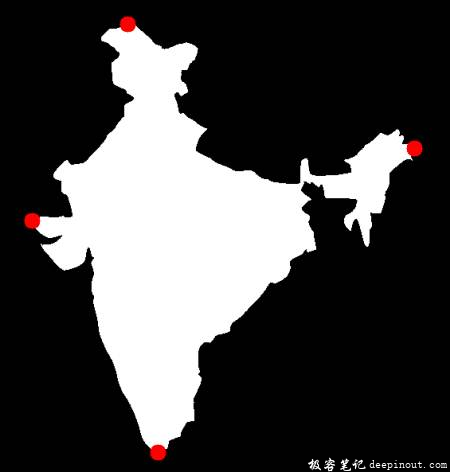# OpenCV-Python轮廓属性

(注意：质心，面积，周长等也属于此类，但我们在上一章中已经看到了)

## OpenCV轮廓长宽比

Aspect \; Ratio = \frac{Width}{Height}

x,y,w,h = cv.boundingRect(cnt)
aspect_ratio = float(w)/h


## OpenCV轮廓范围

Extent = \frac{Object \; Area}{Bounding \; Rectangle \; Area}

area = cv.contourArea(cnt)
x,y,w,h = cv.boundingRect(cnt)
rect_area = w*h
extent = float(area)/rect_area


## OpenCV轮廓固实性

Solidity = \frac{Contour \; Area}{Convex \; Hull \; Area}

area = cv.contourArea(cnt)
hull = cv.convexHull(cnt)
hull_area = cv.contourArea(hull)
solidity = float(area)/hull_area


## OpenCV轮廓等效直径

Equivalent \; Diameter = \sqrt{\frac{4 \times Contour \; Area}{\pi}}

area = cv.contourArea(cnt)
equi_diameter = np.sqrt(4*area/np.pi)


## OpenCV轮廓方向

(x,y),(MA,ma),angle = cv.fitEllipse(cnt)


## OpenCV轮廓遮罩和像素点

mask = np.zeros(imgray.shape,np.uint8)


## OpenCV轮廓最大值，最小值及其位置

min_val, max_val, min_loc, max_loc = cv.minMaxLoc(imgray,mask = mask)


## OpenCV轮廓平均颜色或平均强度

mean_val = cv.mean(im,mask = mask)


## OpenCV轮廓极端点

leftmost = tuple(cnt[cnt[:,:,0].argmin()])
rightmost = tuple(cnt[cnt[:,:,0].argmax()])
topmost = tuple(cnt[cnt[:,:,1].argmin()])
bottommost = tuple(cnt[cnt[:,:,1].argmax()])• 回顶